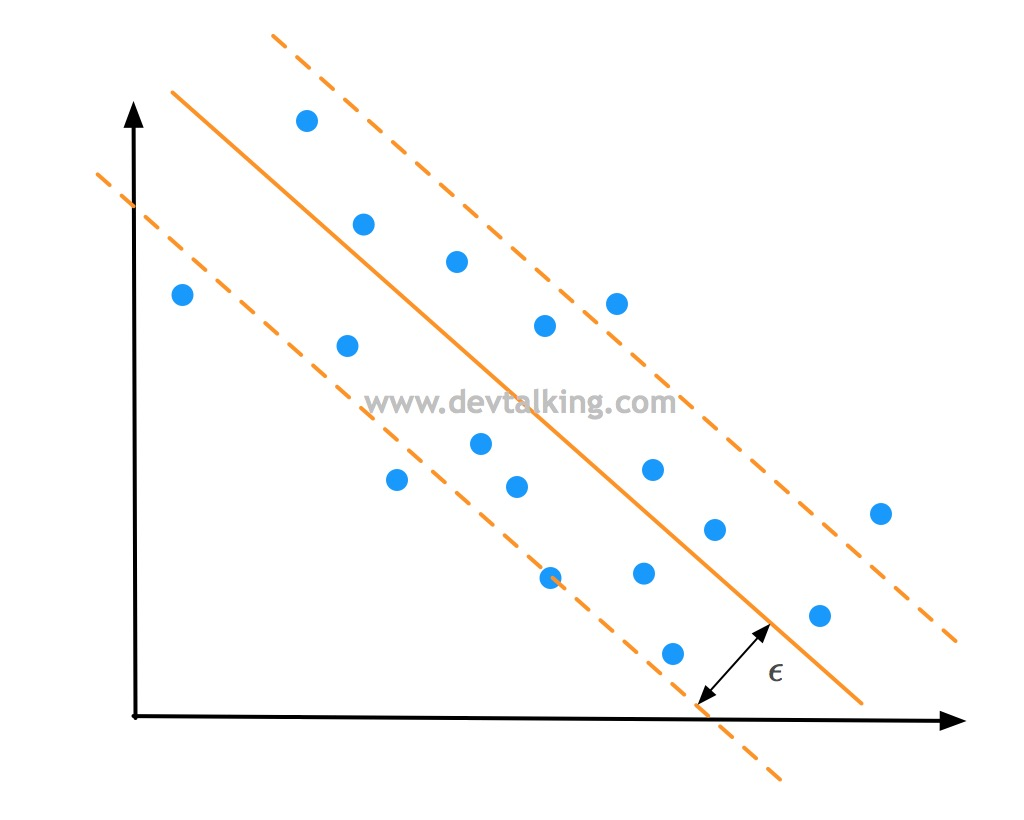SVM处理非线性问题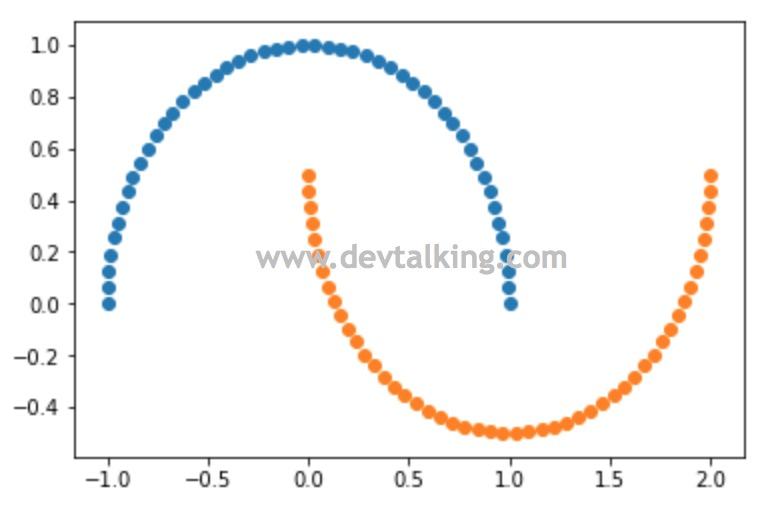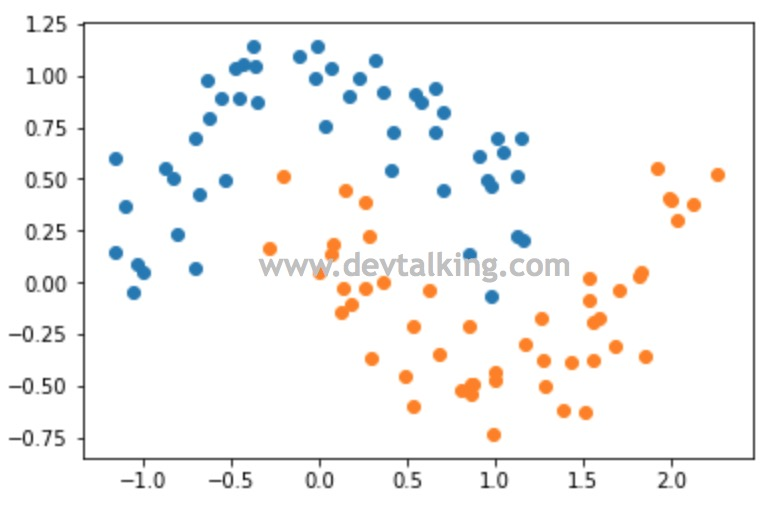• 多项式自然要用到之前讲过的PolynomialFeatures类。
• 数据归一化需要用到StandardScaler类。
• 同样需要用到Pipeline将多个过程封装在一个函数里，所以要用到Pipeline类。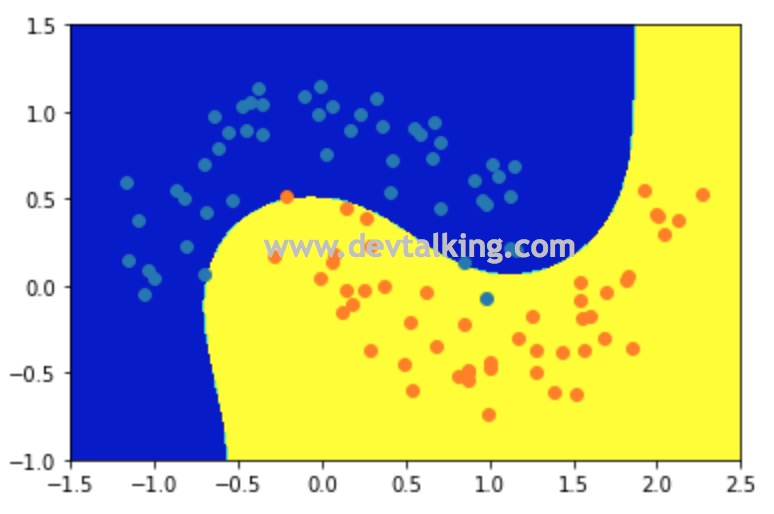核函数（Kernel Function）

什么是核函数

$$K(x,y)=(x \cdot y + 1)^2$$

$$K(x,y)=(\sum_{i=1}^nx_iy_i + 1)$$

$$K(x,y)=\sum_{i=1}^n(x_i^2)(y_i^2) + \sum_{i=2}^n\sum_{j=1}^{i-1}(\sqrt 2 x_ix_j)(\sqrt 2 y_iy_j) + \sum_{i=1}^n(\sqrt 2x_i)(\sqrt 2y_i) + 1$$

• 从$\sum_{i=1}^n(x_i^2)(y_i^2)$可以分析出共有$(x_n^2,…,x_1^2)$这么多项。
• 从$\sum_{i=2}^n\sum_{j=1}^{i-1}(\sqrt 2 x_ix_j)(\sqrt 2 y_iy_j)$可以分析出共有$(\sqrt 2x_nx_{n-1},…,\sqrt 2x_2x_1)$这么多项。
• 从$\sum_{i=1}^n(\sqrt 2x_i)(\sqrt 2y_i)$可以分析出共有$(\sqrt 2x_n,…,\sqrt 2x_1)$

$$x’= (x_n^2,…,x_1^2,\sqrt 2x_nx_{n-1},…,\sqrt 2x_2x_1,\sqrt 2x_n,…,\sqrt 2x_1,1)$$

$$K(x,y) = (x \cdot y + c)^d$$

$d$ 代表多项式中的degree，$c$代表Soft Margin SVM中的$C$，是两个超参数。

通过核函数方式使SVM处理非线性问题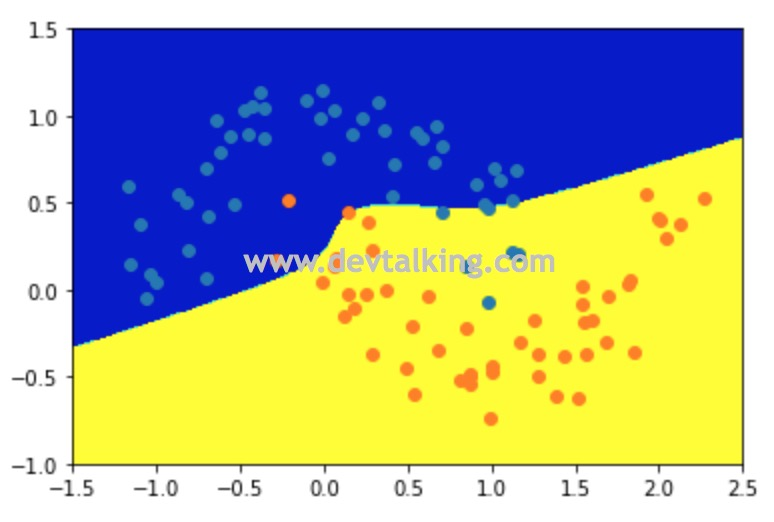Scikit Learn中使用了多项式核函数的SVM的类是SVC，它的kernel参数就表示要使用哪种核函数，上面示例中传入的poly就表示多项式核函数。

高斯核函数

• 高斯核函数。
• 径向基函数。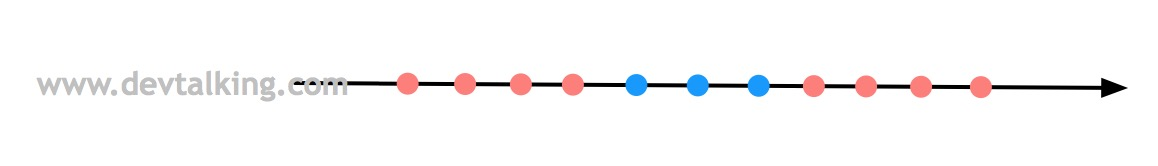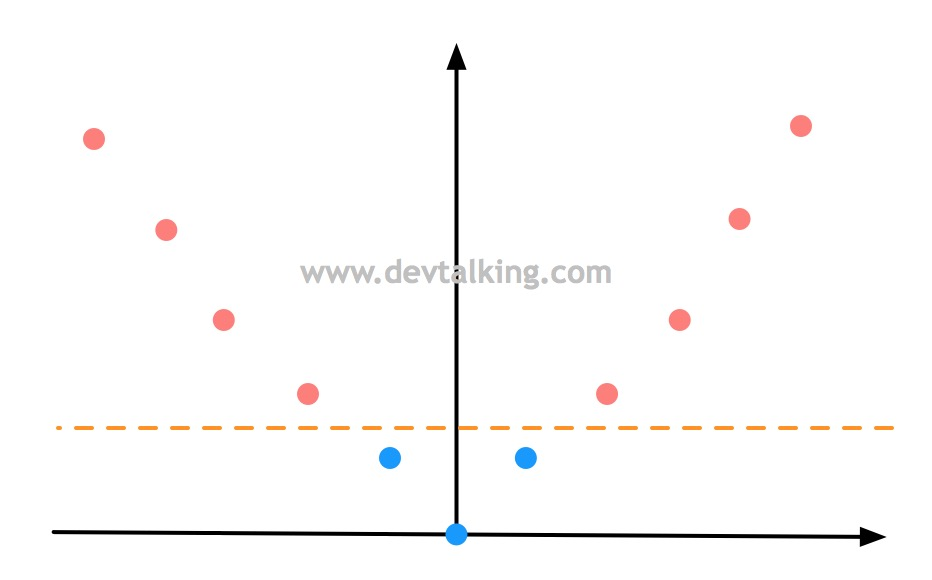$$K(x, y) = e^{-\gamma||x-y||^2}$$

$$x \to (e^{-\gamma||x-l_1||^2}, e^{-\gamma||x-l_2||^2})$$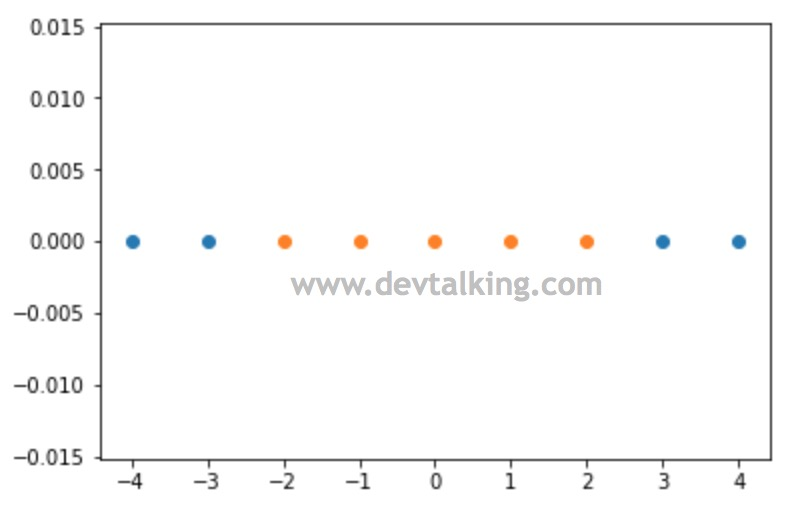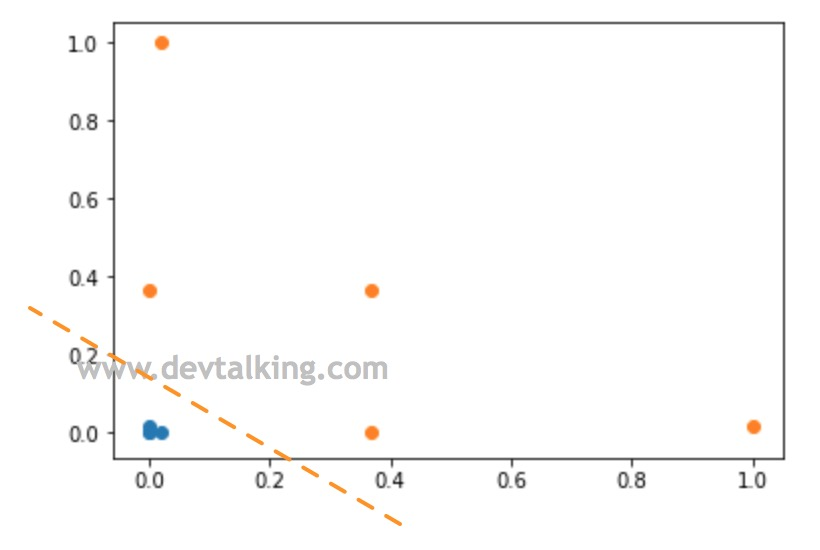高斯核函数中的Gamma

$$f(x) = \frac 1 {\sigma \sqrt {2 \pi}} e^{-\frac {(x-\mu)^2} {2\sigma^2}}$$

• 高斯函数$e$前的系数是$\frac 1 {\sigma \sqrt {2 \pi}}$，$e$指数的系数是$-\frac 1 {2\sigma^2}$。
• 高斯核函数$e$前的系数是1，$e$指数的系数是$-\gamma$。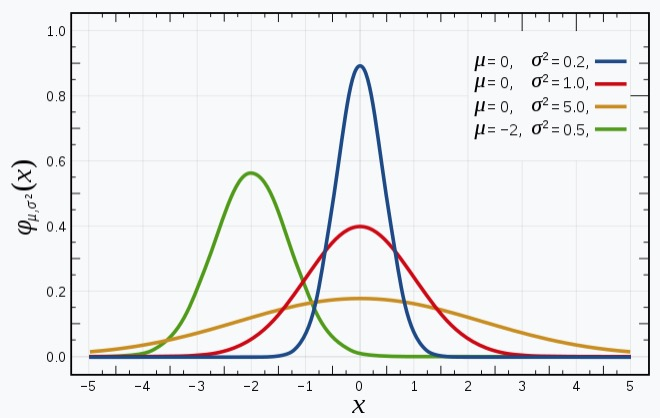• 高斯分布曲线的形状都是相似的钟形图。
• $\mu$决定分布图中心的偏移情况。
• $\sigma$决定分布图峰值的高低，或者说钟形的胖瘦程度。

• 高斯函数中$\sigma$越大、高斯分布峰值越小。$\sigma$越小、高斯分布峰值越大。
• 高斯核函数中$\gamma$越大、高斯分布峰值越大，既钟形越窄。$\gamma$越小、高斯分布峰值越小，既钟形越宽。

Scikit Learn中的RBF SVM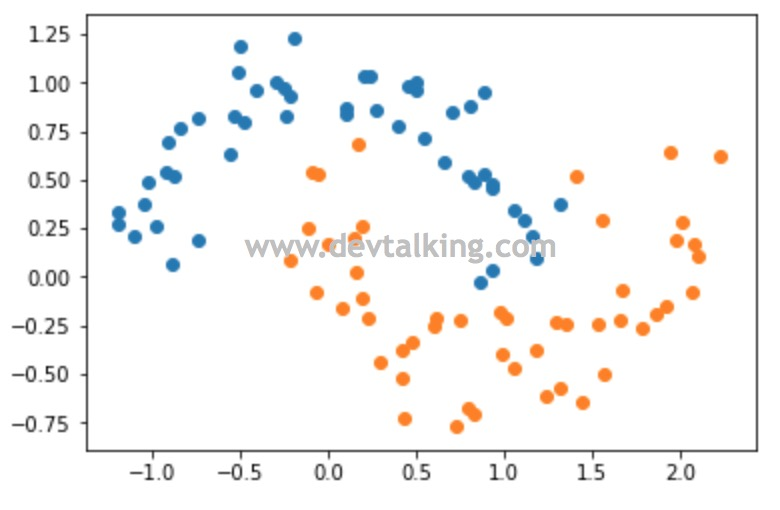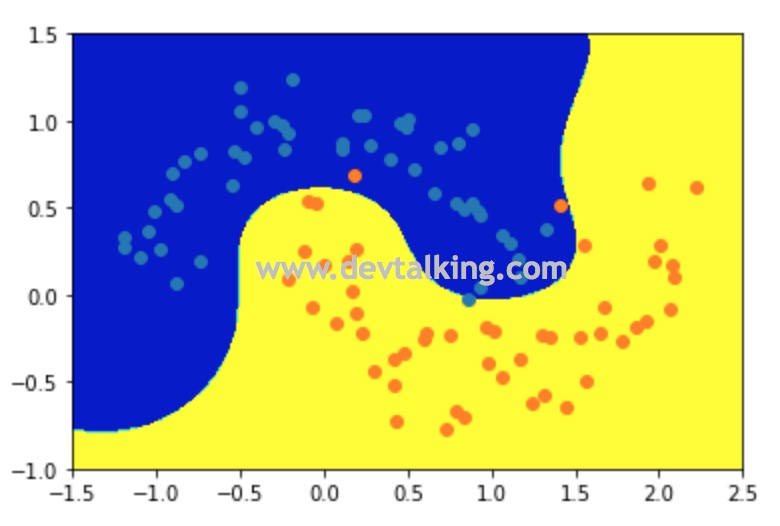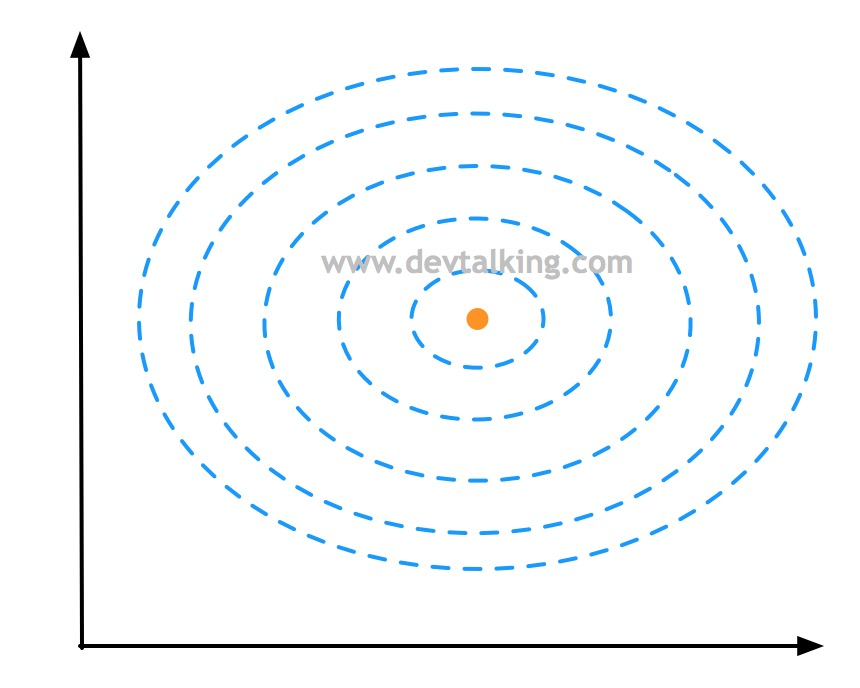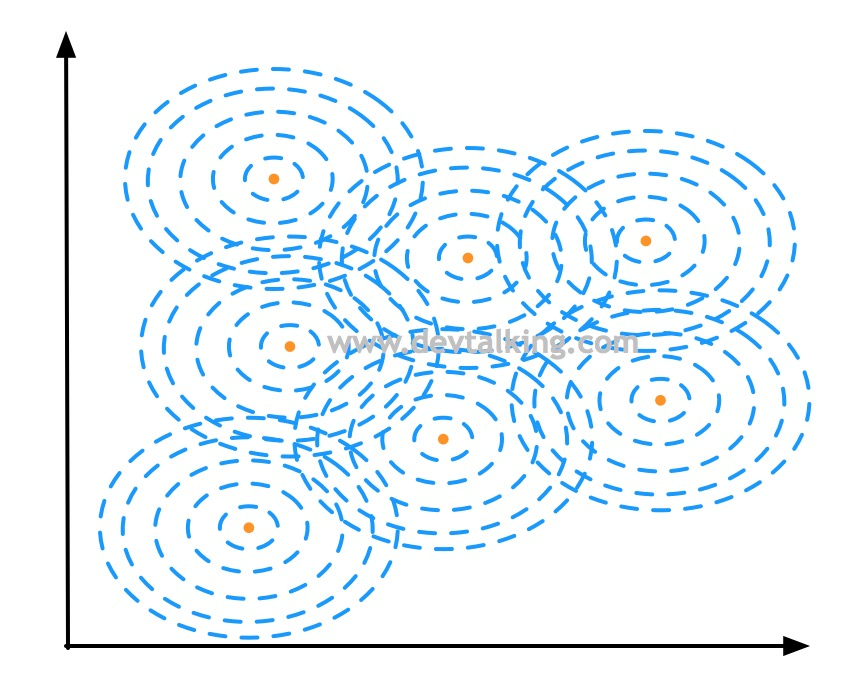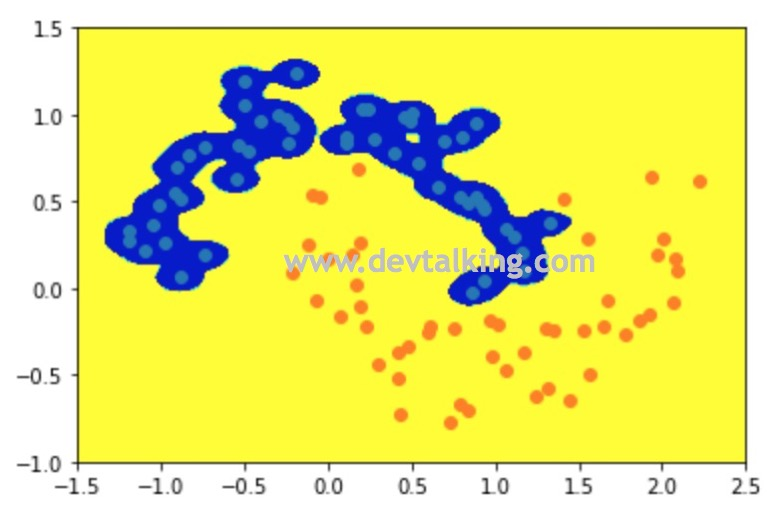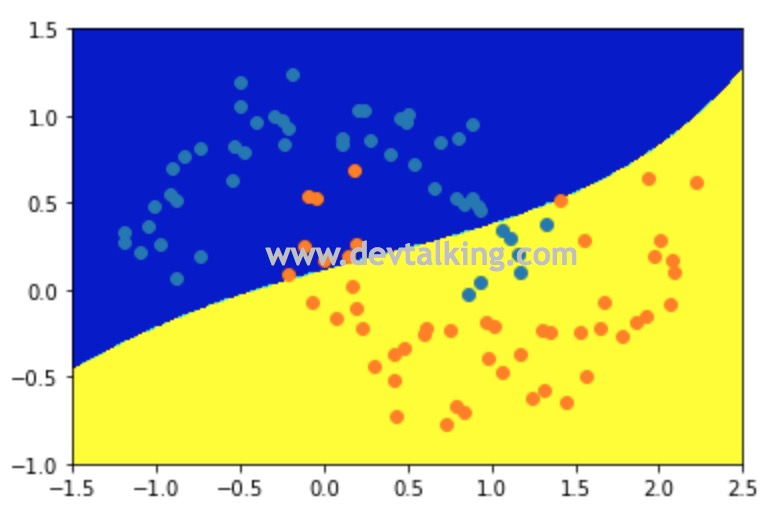SVM解决回归问题

SVM解决回归问题的思路和解决分类问题的思路正好是相反的。我们回忆一下，在Hard Margin SVM中，我们希望在Margin区域中一个样本点都没有，即便在Soft Margin SVM中也是希望Margin区域中的样本点越少越好。